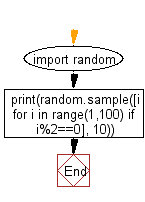﻿ Python: Randomly generate a list with 10 even numbers between 1 and 100 inclusive - w3resource# Python: Randomly generate a list with 10 even numbers between 1 and 100 inclusive

## Python Basic - 1: Exercise-81 with Solution

Write a Python program to randomly generate a list with 10 even numbers between 1 and 100 inclusive.

Note: Use random.sample() to generate a list of random values.

Sample Solution:

Python Code:

``````import random
print(random.sample([i for i in range(1,100) if i%2==0], 10))
``````

Sample Output:

```[4, 22, 8, 20, 24, 12, 30, 98, 28, 48]
```

Pictorial Presentation:Flowchart:Python Code Editor:

Have another way to solve this solution? Contribute your code (and comments) through Disqus.

What is the difficulty level of this exercise?

Test your Programming skills with w3resource's quiz.

﻿

## Python: Tips of the Day

What is the difference between Python's list methods append and extend?

append: Appends object at the end.

```x = [1, 2, 3]
x.append([4, 5])
print (x)
```

Output:

```[1, 2, 3, [4, 5]]
```

extend: Extends list by appending elements from the iterable.

```x = [1, 2, 3]
x.extend([4, 5])
print (x)
```

Output:

```[1, 2, 3, 4, 5]
```

Ref: https://bit.ly/2AZ6ZFq